# Force

In classical physics, force is defined as the rate of change of momentum with respect to time (i.e. the derivative of momentum with respect to time.) In many everyday scenarios where the speed of a body is much less than that of light (non-relativistic), and the mass of the body is constant, it simplifies to the product of mass and acceleration. In other words, how hard you push on something determines how rapidly you change its speed. Of course, the heavier it is, the more it resists this change.

Using mathematics, force may be defined as the time rate of change of momentum of a body. The SI unit of force is the newton and the US customary system unit is the pound.

Classically, the momentum of an object is given by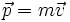and acceleration relates to force via Newton's Second Law aswhen mass can be assumed to be constant. More generally it is prescribed as. In these expressions, F stands for the total vector sum of all forces, m for the mass of the object, a for its acceleration expressed as a vector, p stands for momentum vector and v for velocity vector.

## Force in Relativity

In special relativity, these prescriptions must be modified so as to be Lorentz invariant, which among other things, means that all inertial reference frames stand on equal footing and have the same prescription for all physical and dynamical quantities, though observers in different inertial reference frames will measure different values for many of them, each observer being correct for his own frame. The relationship between force and acceleration in special relativity can be derived using Newton's second law using relativistic momentum: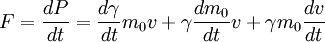Since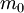is the rest mass and therefore constant, it's derivative is zero. The derivative of speed is acceleration Hence,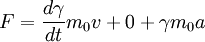Now consider a force that is parallel to motion and perpendicular to motion.

### Force parallel to motion

To differentiate the Lorentz factor,, use the chain rule:Differentiating the Lorentz factor with respect to speed, we find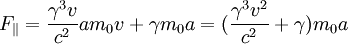After a bit of rearranging, we find that the term in the bracket simplifies to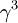. Hence we find for a force acting parallel to an objects motion is: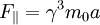### Force perpendicular to motion

A force perpendicular to an objects motion cannot do any work on that object. Hence the kinetic energy of the object and so its speed must remain constant. Therefore,must be constant and its derivative zero. Hence we derive the expression for a perpendicular force as: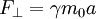### Direction of Force and Acceleration

Rearranging the two forces for acceleration, we find: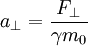Note that since all forces can be resolved into a component parallel and perpendicular to motion, and for all velocities except zero, the parallel and perpendicular accelerations will be different. Hence we can conclude that in the general case (where the force is not completely parallel or perpendicular to motion) that the acceleration vector will have a different direction to the force vector.

## Fundamental Forces

There are four known fundamental types of forces occurring in nature:

Electromagnetic force
The force that acts on objects with electric charge.
Gravitational force
The force that attracts any two objects with mass.
Strong force
The force that keeps atomic nuclei together, as well as binding quarks together to form protons and neutrons.
Weak force
This force is involved in beta decay, in which a neutron in an atomic nucleus is changed to a proton, emitting an electron and an electron anti-neutrino.

It should be noted that the latter two forces have an extremely short range (on the order of femtometers), and that a classical (Newtonian or relativistic) description of these forces is not possible. They can only be described using quantum field theory, a relativistic version of quantum mechanics.